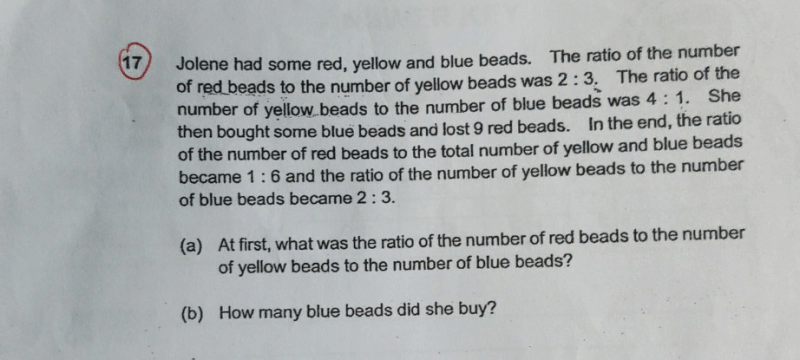# QuestionR : Y : B

2 : 3 (multiply by 4)

4 : 1 (multiply by 3)

8 : 12 : 3 (answer to part (a))

Let x be the amount of blue beads she bought.

Then, (8 units – 9) : (15 units + x) = 1: 6 —- Eqn (1) and

12 units : 3 units + x = 2: 3 —– Eqn (2)

From Eqn (2),

12 units * 3 = (3units + x)*2

36 units = 6 units + 2x

x = 15 units

Therefore, (8 units – 9) * 6 = 30 units -> 48 units – 54 = 30 units

18 units = 54, 1 unit = 3, x = 15 units = 45.

0 Replies 0 Likes

a) 8:12:3

b) Final ratio of red: yellow:blue is  5:12:18

Number of blue bought = 9/ (8-5)  x (18-3) = 45

0 Replies 0 Likes